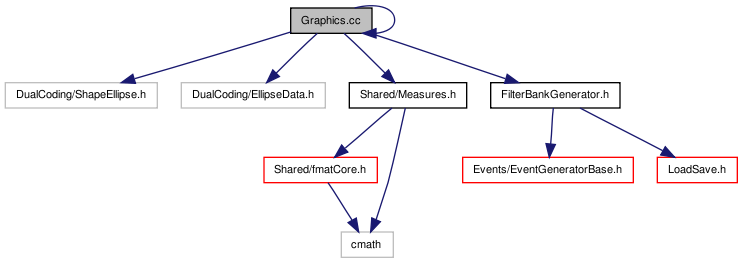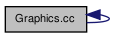# Graphics.cc File Reference

`#include "DualCoding/ShapeEllipse.h"`
`#include "DualCoding/EllipseData.h"`
`#include "Graphics.h"`
`#include "Shared/Measures.h"`
`#include "FilterBankGenerator.h"`
Include dependency graph for Graphics.cc:This graph shows which files directly or indirectly include this file:Go to the source code of this file.

## Defines

#define CHKBOUNDS(p, ERR_F)
empty definition of macro for checking for any exceeding of image's memory boundaries

## Detailed Description

Definition in file Graphics.cc.

## Define Documentation

 #define CHKBOUNDS ( p, ERR_F )
Value:
```{ \
unsigned int rowoffset=(p-img1)-(p-img1)/yInc*yInc; \
if(p<img1 || p>=img1+h*yInc || rowoffset>=w*xInc || rowoffset/xInc*xInc!=rowoffset) { \
cout << "Graphics Bad draw! line:" << __LINE__ << " frame=" << (gen!=NULL?gen->getFrameNumber():0) << ' '  /*<< (void*)p << '-' << (void*)img1 << '='*/ << (int)(p-img1) << " w=" << w << " xInc=" << xInc << " h=" << h << " yInc=" << yInc << endl; \
ERR_F; \
} \
}
```

empty definition of macro for checking for any exceeding of image's memory boundaries

Definition at line 12 of file Graphics.cc.

Referenced by Graphics::drawLine(), and Graphics::drawRect().

 Tekkotsu v5.1CVS Generated Mon May 9 04:58:56 2016 by Doxygen 1.6.3